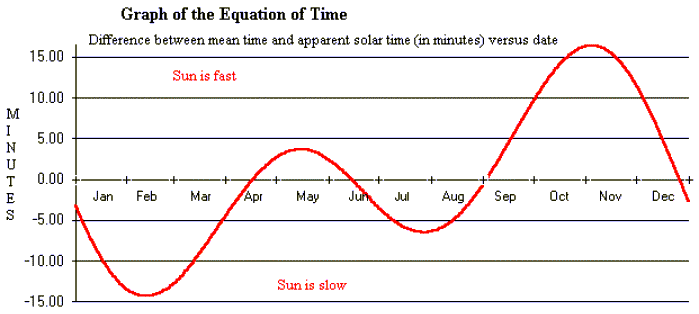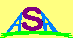### THE EQUATION OF TIME

The time that you read from a sundial is not the same time you read from a watch or clock. There are two reasons for this difference. One is due to our use of zonal time (times which are referred to fixed longitudes which are 15 degrees apart). For instance, Australian Eastern Standard Time (AEST) is a time referred to a longitude of 150o east of the prime meridian (that line of longitude that passes through the Royal Greenwich Observatory in England), and this gives a time which is 10 hours ahead of Universal Time (UT), a time which is based on that prime meridian (ie at 0o longitude).

Now Sydney is quite close to 150o longitude, and so we would expect the Sun to pass across its central meridian (ie the north-south line) quite close to noon (clock time). However, Melbourne is located around 145o longitude, and this corresponds to (145-150)/15 hours or -20 minutes, and so we might expect the Sun to cross the local meridian in Melbourne at around 11:40.

However, there is a second effect which displaces the actual time of solar central meridian passage or CMP from the times listed above. This effect is called the "Equation of Time", and it is the time in minutes by which the time of CMP is displaced from mean (or average) solar noon (clock time).The equation of time is due to two phenomena: the tilt of the Earth's rotational axis (23.5o) and the fact that the Earth moves around the Sun in an elliptical orbit. When a planet moves in an elliptical orbit, it moves faster when it is at perihelion than when it is at aphelion. This appears to surface inhabitants as if the Sun moves around the Earth faster at perihelion (currently January) and slower at aphelion (currently July). The tilt of the Earth's axis makes the Sun appear to cross one's central meridian at different times than if the axis were perpendicular to the orbital plane. These two effects add to produce the equation of time (which has values shown by the above graph).

When the Sun is fast, CMP will occur earlier. When it is slow, CMP will occur later. So in Melbourne, apparent solar noon will occur around 11:24 AEST in the first week of November, and around 11:54 in the middle of February. If daylight saving is in operation, clock time is displaced another 60 minutes (one hour) from Sun time. This means that noon in the first week of November will occur at a clock time of 12:24 AEDT (Australian Eastern Daylight Time).

The actual equation of time, which is mathematically defined as (apparent solar time - mean solar time), varies slightly from year to year due to small variations in the Earth's eccentricity and obliquity and in the time of the solstices and equinoxes, but for a century either side of the year 2000 it may be approximated (to an accuracy of better than one percent) by the formulae:

EoT(minutes) = 9.87 sin(2Bo) - 7.67 sin(Bo + 78.7o)
where Bo = 360 (N - 81) / 365
and N is the day of the year (eg N=1 on Jan 01 and N=33 on Feb 02)

Note that the equation of time also affects sunrise and sunset times as well as the CMP time.Australian Space Academy